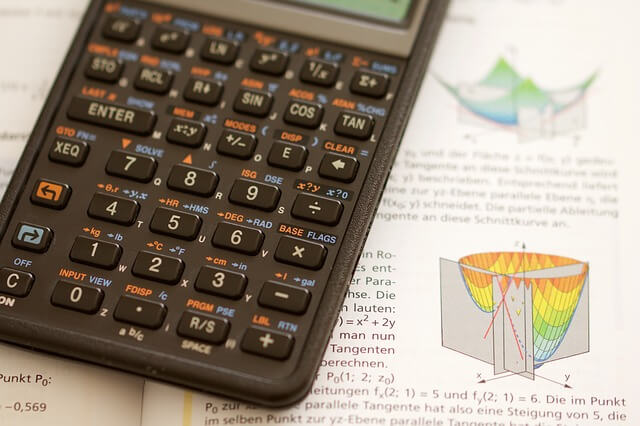### What is mean, mode, median, variance and standard deviation?

In Data Science, we come across terms like mean, mode, median, variance, standard deviation and many more. These arithmetic functions are very useful for solving of data science problems. Let us understand them in some detail.

## What is mean?

Mean is the average of the numbers. It is basically the sum of all the numbers, divided by how many numbers are there.

Example: We have numbers as follows: 2 ,3 ,4 and 7.
First step: sum of all the numbers: 2 + 3 + 4 + 7 = 16

Second step: total numbers in the series: 4

Third step: Divide the sum of all numbers by total numbers in the series: 16 / 4 = 4

Thus we get the mean as 4.Mean, Mode. Median, Variance and Standard Deviation

## What is mode?

The number which appears the most often in a given set of numbers is called mode.

Example: (4, 5, 4, 6, 4, 5, 4, 5, 6, 4)

In the above series of numbers, we have number 4 as the mode as it is occurring the maximum time that is 5 times.

## What is median?

The Median is the middle of a sorted list of numbers.

For this we will have two examples as we will come across both of them frequently when trying to find out the median.

Example:1

list of numbers: (3, 6, 1, 2, 4, 5, 7)

First we shall sort them in order: (1, 2, 3, 4, 5, 6, 7)

Here we get 4 as the median as it is exactly in the middle.

Example:2

What if we add one more number to the list of numbers. We don't get a single number in the middle, but we get a pair of middle numbers. Lets see how to solve it.

list of numbers: (3, 6, 1, 2, 4, 5, 7, 8)

First we shall sort them in order: (1, 2, 3, 45, 6, 7, 8)

Here we get a pair of middle numbers 4 and 5. To find the exact middle we add both the numbers and divide it by 2.

Median = ( 4 + 5 ) / 2 = 4.5

So the median is 4.5

## What is variance?

Variance is related to both mean and standard deviation. Variance can be defined as the average of the squared differences from the mean.

Lets see it step by step:
1. First we work out the mean. Mean as you know is the average of the numbers.
2. Then for each number: subtract it from the mean and square the result.
3. Then work out the average of those difference.
Example: Suppose we have height of different buildings in meters as: ( 10, 15, 20, 10, 25 )

First we work out the mean: ( 10, 15, 20, 10, 25 ) / 5 = 16
Then we work out the difference of each number from mean, square it and then average the result. The final result is the Variance.

Variance = ( 10 - 16 )^2 + ( 15 - 16 )^2 + ( 20 - 16 )^2 + ( 10 - 16 )^2 + ( 25 - 16 )^2 / 5
=  36 + 1 + 16 + 1 + 81 / 5
= 135 / 5
=  27

## What is Standard Deviation?

The Standard Deviation is just the square root of Variance.

So it will be : Square-root ( 27 ) = 5.196

Standard deviation is very useful. Now we can come to know the height of the buildings which are within one standard deviation (5.196 m) of the mean.

With this we will come to know which buildings are having normal heights and which buildings are too large or too small.

Hope you got to know some of the arithmetical terms which we will be using frequently in solving the data science problems.